## Determine Compressibility Factor with Present of CO2 and H2SThis example will demonstrate how to determine the compressibility factor (z) for gas with CO2 and H2S. As it is described in the article,Determine Compressibility of Gases , it states that gas with CO2 and H2S must be corrected. This is similar to a normal method for determining z-factor but it is required some correction. Please follow the steps below;

Gas component is shown in Table 1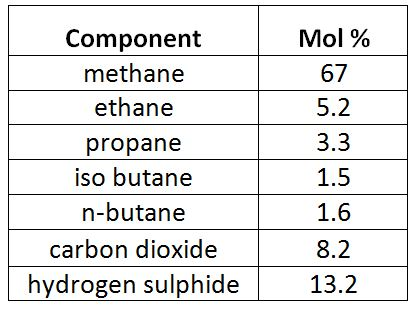Table 1 – Gas Component

Reservoir pressure = 6,000 psia

Reservoir temperature = 190 F Continue reading

## Determine Compressibility of GasesThis article will demonstrate how to determine gas compressibility by using simplified equation of state.

At a low pressure condition, gas similarly behaves like ideal gas and the equation for the ideal gas law is listed below;

# PV = nRT

Where;

P = pressure

V = gas volume

T = absolute temperature

n = number of moles of gas

R = gas constant

Gas constant (R) is different because it depends on the unit system used in the calculation. The R in the different unit system is demonstrated in the below table.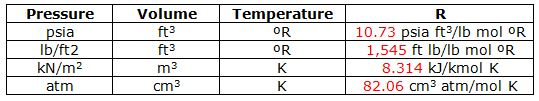Table 1 – Gas Constant (R) at Different Unit

## Volumetric Method To Estimate Volume In Place and ReservesVolumetric is a method to estimate fluid in reservoir based on volume of pore space in a rock and water saturation.

Volume of Oil Initially In Place (OIIP)

To estimate oil initially volume in place, the following formula is a volumetric calculation for oil.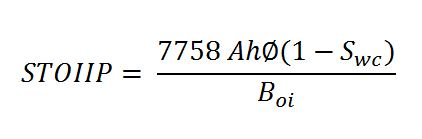Where;

STOIIP = stock tank oil in place, stb

A= area, acre

h = reservoir thickness, ft

ɸ = rock porosity, %

Swc =connate water saturation, %

Boi = oil formation volume factor, rb/stb

Note: the stock tank condition is a standard surface condition of oil and gas at 60F and 14.7 psia. Continue reading

## Material Balance for Gas Reservoir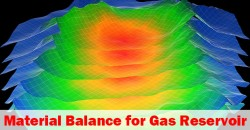For gas reservoirs, the material balance concept can be applied to determine gas in place and expected gas reservoir reserve.

Gas Production = Expansion of Free Gas In Reservoir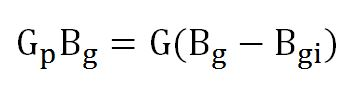Assumption:

• Dry gas reservoir
• No external energy support like water drive.

Where;

Gp = gas production (std cu-ft)

Bg = gas formation volume factor (res cu-ft/std cu-ft)

G = gas in place (std cu-ft)

Bgi = initial formation volume factor (res cu-ft/std cu-ft)

Gas formation volume factor (Bg)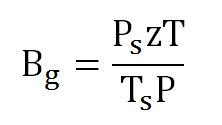Continue reading

## Material Balance for a Water Drive Mechanism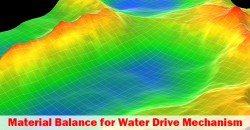This article will demonstrate a material balance equation in a natural water drive mechanism.  A full material balance equation is shown below: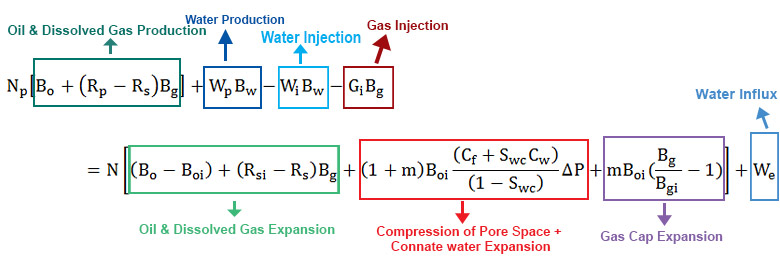Figure 1 – Full Material Balance Equation

Assumptions

• Reservoir pressure above the bubble point (Pb). Above a bubble point, Rs = Rsi.
• No water injection
• No gas injection
• No gas cap
• Neglect formation and connate water compressibility (Cf and Cw have little effect for a gas cap drive mechanism.)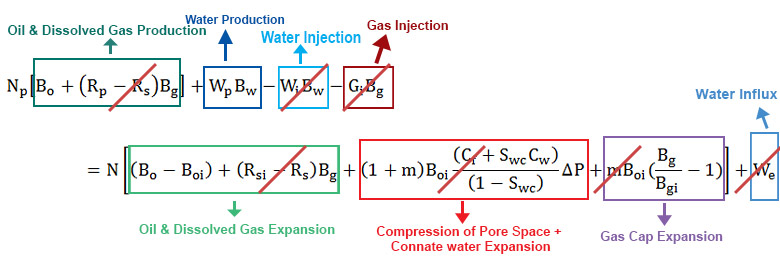Figure 2 – Material Balance Equation with Assumption for a Natural Water Drive Mechanism